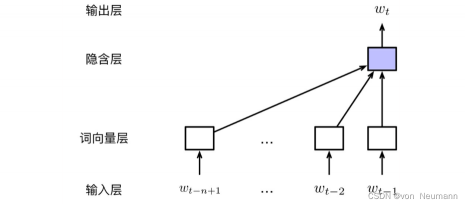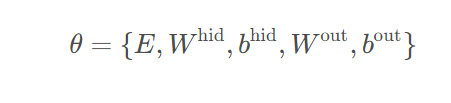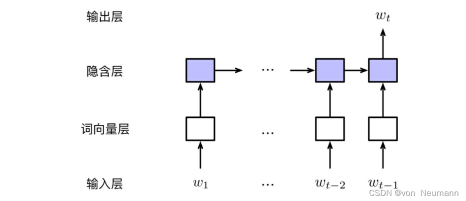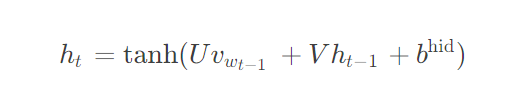《自然语言处理从入门到应用——自然语言处理的语言模型（Language Model，LM）》中介绍了语言模型的基本概念，以及经典的基于离散符号表示的N元语言模型（N-gram Language Model）。从语言模型的角度来看，N元语言模型存在明显的缺点。首先，模型容易受到数据稀疏的影响，一般需要对模型进行平滑处理；其次，无法对长度超过N的上下文依赖关系进行建模。神经网络语言模型（Neural Network Language Model）在一定程度上克服了这些问题。一方面，通过引入词的分布式表示，也就是词向量，大大缓解了数据稀疏带来的影响；另一方面，利用更先进的神经网络模型结构（如循环神经网络、Transformer等），可以对长距离上下文依赖进行有效的建模。正因为这些优异的特性，加上语言模型任务本身无须人工标注数据的优势，神经网络语言模型几乎已经替代N元语言模型，成为现代自然语言处理中最重要的基础技术之一；同时，也是自然语言预训练技术的核心。本文将从最基本的前馈神经网络语言模型出发，介绍如何在大规模无标注文本数据上进行静态词向量的预训练；然后，介绍基于循环神经网络的语言模型，通过引入更丰富的长距离历史信息，进一步提升静态词向量的表示能力。

P(w_t | w_{1:t-1}) = P(w_t | w_{t-n+1:t-1})• 输入层：模型的输入层由当前时刻t的历史词序列 w_{t-n+1:t-1} 构成，主要为离散的符号表示。在具体实现中，既可以使用每个词的独热编码（One-Hot Encoding），也可以直接使用每个词在词表中的位置下标

• 词向量层：词向量层将输入层中的每个词分别映射至一个低维、稠密的实值特征向量。词向量层也可以理解为一个查找表（Look-up Table），获取词向量的过程，也就是根据词的索引从查找表中找出对应位置的向量的过程：

x = [v_{w_{t-n+1}};v_{w_{t-n+2}}; … ;v_{w_{t-1}}]

式中，v_w \in R^d表示词wd维词向量（ d \ll |V| ∣ V ∣为词表大小）；x \in R^{(n-1) d}表示历史序列中所有词向量拼接之后的结果。若定义词向量矩阵为E \in R^{d \times        |V|}，那么v_w即为E中与w对应的列向量，也可以表示为Ew的独热编码w_e之间的点积。

• 隐藏层：模型的隐藏层对词向量层x进行线性变换与激活。令W^{hid} \in R^{m \times (n-1)d}为输入层到隐藏层之间的线性变换矩阵,b^{hid} \in R^m为偏置项，m为隐藏层维度。隐藏层可以表示为：

h = f(W^{hid} x + b^{hid})

式中，f是激活函数。常用的激活函数有Sigmoid、tanh和ReLU等。

• 输出层：模型的输出层对h做线性变换，并利用Softmax函数进行归一化，从而获得词表V空间内的概率分布。令W^{out}为隐藏层到输出层之间的线性变换矩阵，相应的偏置项为b^{out}。输出层可由下式计算：

y = Softmax(W^{out} x + b^{out})

综上所述，前馈神经网络语言模型的自由参数包含词向量矩阵E，词向量层与隐藏层之间的权值矩阵W^{hid}及偏置项b^{hid}，隐藏层与输出层之间的权值矩阵W^{out}与偏置项b^{out}，可以记为：• 输入层：与前馈神经网络语言模型不同，由于模型不再受限于历史上下文的长度，所以此时输入层可由完整的历史词序列构成，即w_{1:t-1}
词向量层：与前馈神经网络语言模型类似，输入的词序列首先由词向量层映射至相应的词向量表示。那么，在t时刻的输入将由其前一个词w_{t-1}的词向量以及t − 1时刻的隐含状态h_{t-1}组成。令w_0为句子起始标记（如：＜bos＞）， h_0为初始隐含层向量（可使用0向量），则t时刻的输入可以表示为：

x_t = [v_{w_{t-1}} ; h_{t-1}]

• 隐含层：隐含层的计算与前馈神经网络语言模型类似，由线性变换与激活函数构成。

h_t = tanh(W^{hid} x_t + b^{hid})

式中W^{hid} \in R^{m \times (d+n)}, b^{hid} \in R^mW^{hid}实际上由两部分构成，即W^{hid} = [ U; V]U \in R^{m \times d}、V \in R^{m \times m} 分别是v_{w_{t-1}} 、 h_{t-1}与隐含层之间的权值矩阵。为了体现循环神经网络的递归特性，在书写时常常将两者区分开：• 输出层：最后，在输出层计算t时刻词表上的概率分布：

y = Softmax(W^{out} x + b^{out})

式中，W^{out} \in R^{|V| \times m}

 车万翔, 崔一鸣, 郭江. 自然语言处理：基于预训练模型的方法[M]. 电子工业出版社, 2021.
 邵浩, 刘一烽. 预训练语言模型[M]. 电子工业出版社, 2021.
 何晗. 自然语言处理入门[M]. 人民邮电出版社, 2019
 Sudharsan Ravichandiran. BERT基础教程：Transformer大模型实战[M]. 人民邮电出版社, 2023
 吴茂贵, 王红星. 深入浅出Embedding：原理解析与应用实战[M]. 机械工业出版社, 2021.